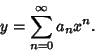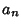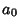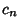## Frobenius Method

Ifis an ordinary point of the Ordinary Differential Equation, expandin a Taylor Series about, letting(1)

Plugback into the ODE and group the Coefficients by Power. Now, obtain a Recurrence Relation for theth term, and write the Taylor Series in terms of thes. Expansions for the first few derivatives are(2)(3)(4)

Ifis a regular singular point of the Ordinary Differential Equation,(5)

solutions may be found by the Frobenius method or by expansion in a Laurent Series. In the Frobenius method, assume a solution of the form(6)

so that(7)(8)(9)

Now, plugback into the ODE and group the Coefficients by Power to obtain a recursion Formula for theth term, and then write the Taylor Series in terms of thes. Equating theterm to 0 will produce the so-called Indicial Equation, which will give the allowed values ofin the Taylor Series.

Fuchs's Theorem guarantees that at least one Power series solution will be obtained when applying the Frobenius method if the expansion point is an ordinary, or regular, Singular Point. For a regular Singular Point, a Laurent Series expansion can also be used. Expandin a Laurent Series, letting(10)

Plugback into the ODE and group the Coefficients by Power. Now, obtain a recurrence Formula for theth term, and write the Taylor Expansion in terms of thes.

Arfken, G. Series Solutions--Frobenius' Method.'' §8.5 in Mathematical Methods for Physicists, 3rd ed. Orlando, FL: Academic Press, pp. 454-467, 1985.